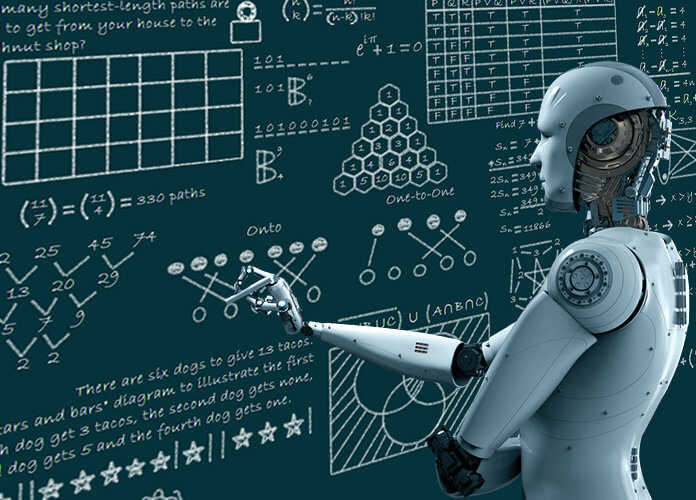Categories

# Machine LearningMachine learning is the one of the most important aspect of artificial intelligence. Machine learning is first coined by Arthur Samuel in the year 1959. Is artificial intelligence and machine learning is the same? No, both are not same. The difference between Machine learning and AI is that machine learning is used in AI.

### Machine learning is a method through which we can feed a lot of data to a machine and make it learn.

AI is a vast of field. Under AI, we have machine learning, NLP, expert systems, image recognition, object detection and deep learning and so on. AI is sorts of process in which we make machines mimic the behaviour of human beings.

### Need for Machine Learning:

Need for Machine learning begins since technical revolution itself. Since technology became the centre of everything, we have been generating an immeasurable amount of data. As per research, we generate around 2.5 quintillion bytes of data, every single data every single day. And it is estimated that by this year 2020, 1.7mb of data will be created every second for every person on earth. So, we are generating a lot of data.

Now you are reading this article from one of the website. That means we are using data. So data is everywhere. The availability of so much of data, it is finally possible to build predictive models that study and analyse complex data to find useful insights and deliver more accurate results.

Top tier companies like Netflix and Amazon are built such machine learning models by using tons of data in order to identify any profitable opportunity and avoid any unwanted risk.

A few reasons machine learning is so important is:

1. Due to excessive production of Data we need to find a method that can be used to structure, analyse and useful insights from data, this is where machine learning comes in. It is used solve problems and find solutions through the most complex task faced by organizations.
2. Other reason is we also needed to improve decision making. By making use of various algorithms, machine learning can be used to make better business decisions. For example, machine learning is used to focus sales. It is used to predict any downfalls any stock market or identify any sort of risk and anomalies.
3. One more reason is Machine learning helps us uncover patterns and trends in data. Finding hidden data and extracting key insights from data is the most important part of machine learning. So by building predictive models and statistical techniques, machine learning allows us to dig beneath the surface and explode the data at a minute scale.

Understanding data and extracting patterns manually takes a lot of time. It will take seven days for us to extract any useful information from data. But if we use machine learning algorithms, we can perform similar computations in less than a second.

1. Another reason is we need to solve complex problems. So from detecting the genes linked to the deadly ALS disease, to building self-driving cars, machine learning is used to solve most complex problems. At present, we also found a way to spot stars which ae 2400 light years away from our planet. All of this is possible through AI, Machine learning, deep learning.

### A computer program is said to learn from experience E with respect to some class of tasks T and performance measure P if its performance at task in T, as measured by P, improves with experience E.

That’s very confusing, so let me just narrow it down to you. In simple terms, machine learning is a subset of artificial intelligence which provides machines the ability to learn automatically and with experience without being explicitly programmed to do so. In the sense, it practices of getting machines to solve problems by gaining the ability to think.

But now you may be thinking how can a machine think or make decisions. Machines are very similar to humans. If you feed a machine a very good amount of data, it will learn how to interpret and process and analyse this data by using machine learning algorithms, it will help you solve world problems.

What happens here is a lot of data is fed to the machine. The machine will train on this data and it builds a predictive model with the help of machine learning algorithms in order to predict
some outcomes.

Now we will discuss a couple of terms which are frequently used in Machine Learning:

Algorithm: A set of rules and statistical techniques used to learn patterns from data. The logic behind a machine learning model is basically machine learning algorithm. Example of machine learning algorithm is linear regression, decision tree or random forest.

Model: Model is the main component of machine learning process. Model is trained by using a Machine learning algorithm.

The Difference between an Algorithm and a Model is that an algorithm maps all the decisions that a model is supposed to take based on the given input in order to get the correct output. The model will use the machine learning algorithms in order to draw useful insights from the input and give you an outcome that is very precise.

Predictor Variable: Predictor variable is a feature(s) of the data that can be used to predict the output.

Response Variable: Response variable is the feature or the output variable that needs to be predicted by using the predictor variable(s).

For example you are trying to predict the height of a person, depending on his weight. Here your predictor variable becomes your weight, because you are using the weight of a person to predict the person’s height.  And here height is the response variable.

Training Data: The machine learning model is built using the training data.

Testing Data: The testing data is used to test the efficiency of the model.